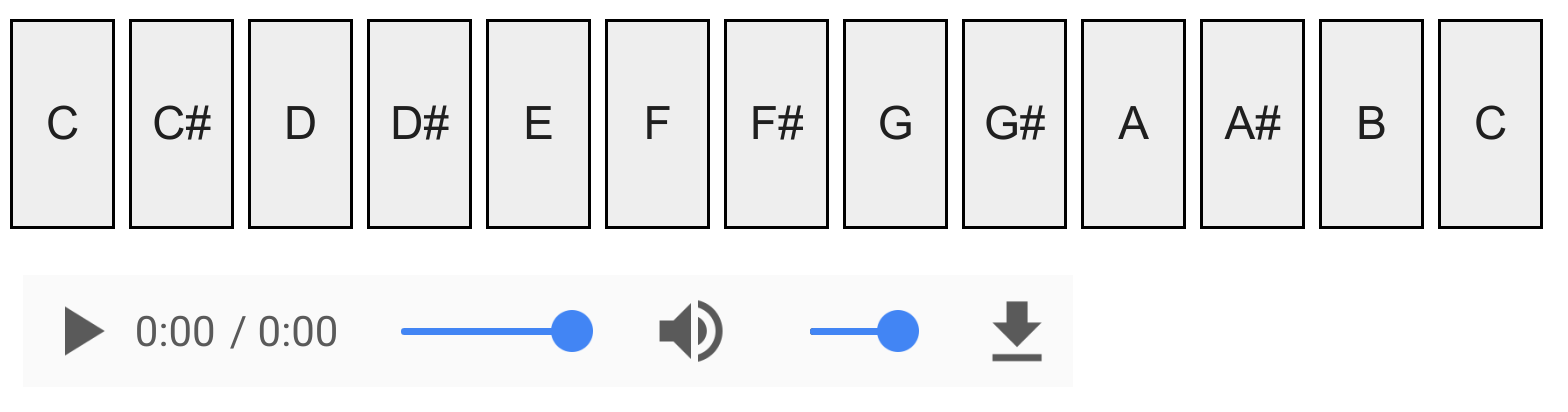This is one of the 100+ free recipes of the IPython Cookbook, Second Edition, by Cyrille Rossant, a guide to numerical computing and data science in the Jupyter Notebook. The ebook and printed book are available for purchase at Packt Publishing.

▶  Text on GitHub with a CC-BY-NC-ND license
▶  Code on GitHub with a MIT license

In this recipe, we will create a small electronic piano in the Notebook. We will synthesize sinusoidal sounds with NumPy instead of using recorded tones.

## How to do it...

1.  We import the modules:

import numpy as np
import matplotlib.pyplot as plt
from IPython.display import (
Audio, display, clear_output)
from ipywidgets import widgets
from functools import partial
%matplotlib inline


2.  We define the sampling rate and the duration of the notes:

rate = 16000.
duration = .25
t = np.linspace(
0., duration, int(rate * duration))


3.  We create a function that generates and plays the sound of a note (sine function) at a given frequency, using NumPy and IPython's Audio class:

def synth(f):
x = np.sin(f * 2. * np.pi * t)
display(Audio(x, rate=rate, autoplay=True))


4.  Here is the fundamental 440 Hz note:

synth(440)5.  Now, we generate the note frequencies of our piano. The chromatic scale is obtained by a geometric progression with the common ratio $$2^{1/12}$$:

notes = 'C,C#,D,D#,E,F,F#,G,G#,A,A#,B,C'.split(',')
freqs = 440. * 2**(np.arange(3, 3 + len(notes)) / 12.)
notes = list(zip(notes, freqs))


6.  Finally, we create the piano with the Notebook widgets. Each note is a button, and all buttons are contained in a horizontal box container. Clicking on one note plays a sound at the corresponding frequency.

layout = widgets.Layout(
width='30px', height='60px',
border='1px solid black')

buttons = []
for note, f in notes:
button = widgets.Button(
description=note, layout=layout)

def on_button_clicked(f, b):
# When a button is clicked, we play the sound
# in a dedicated Output widget.
with widgets.Output():
synth(f)

button.on_click(partial(on_button_clicked, f))
buttons.append(button)

# We place all buttons horizontally.
widgets.Box(children=buttons)## How it works...

A pure tone is a tone with a sinusoidal waveform. It is the simplest way of representing a musical note. A note generated by a musical instrument is typically much more complex. Although the sound contains many frequencies, we generally perceive a musical tone (fundamental frequency).

By generating another periodic function instead of a sinusoidal waveform, we would hear the same tone, but a different timbre. Electronic music synthesizers are based on this idea.

## There's more...

Here are a few references: